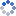加载中…氯化钡和硫酸银
• 博客等级：• 博客积分：0
• 博客访问：91,145
• 关注人气：37
• 获赠金笔：0支
• 赠出金笔：0支
• 荣誉徽章：

## 基础集合论（5）：选择公理、良序化原理、Zorn 引理(2015-11-04 16:06:59)

### 杂谈

良序化原理：对任意集合 A，存在 A 上的一个偏序 <=，使 (A, <=) 是良序集。
前面讨论过，从直觉上看，通过在后面不断加元素的方法，可以从小到大造出所有良序集。为此，我们按一定的顺序从 A 中选元素往后加，希望能把 A 中元素全取遍，这样就搞出了 A 上的一个良序。自然，像之前证明存在从 ω 到无穷集的单射一样，我们需要借助选择公理。然而光有这还不够，“从 A 中选元素往后加，能把 A 中元素全取遍”这种叙述显然不能成为一个正确的证明。为此，我们考虑 A 的所有“按照这样的顺序”（指的是按照选择函数 φ 的顺序，例如 a0=φ(A), a1=φ(A-{a0}), a2=φ(A-{a0, a1})，等等，就像之前证明存在从 ω 到无穷集的单射时所做的一样）排出的良序子集，期望它们的并集就是整个 A 上的良序。
下面开始正式的证明。若 A 为空集，则 (∅, ∅) 就是所要的良序集，因此可设 A 非空。由选择公理，设 φ 是集合 A 的一个选择函数。若集合 B 为 A 的子集，且 B 上有良序 <=_B 满足（注意，它保证了 B 上的良序是“按照选择函数 φ 的顺序”的！），则称良序集 (B, <=_B) 为正规良序集。当然，有时我们也简单地说 B 为正规良序集。
接下来，分几步证明良序化原理。
1.若 (B, <=_B), (B, <='_B) 都是正规良序集，则两个良序 <=_B, <='_B 相同。
（下面称 <=_B, <='_B 为第一良序和第二良序）
事实上，只需证明对 B 中任意元素 a，按两种良序的前段相同（即 s_B(a)=s'_B(a)）就行了。按第一良序对 a 超限归纳，即假设按第一良序，对任意小于 a 的元素 x 都有 s_B(x)=s'_B(x) 。此时先证明集合 s_B(a) 按第二良序仍然是截段（这是因为任取 s_B(a) 中元素 x，以及按第二良序小于 x 的元素 y，由于 x 按两种良序的前段相同，因此按第一良序仍有 y 小于 x，又 x 小于 a，故 y 小于 a，即 y 属于 s_B(a)，这说明 s_B(a) 按第二良序仍然是截段），设 s_B(a) 是 a' 按第二良序的前段，即 s_B(a)=s'_B(a')，按照正规良序集的定义，有2.若 (B, <=_B), (C, <=_C) 都是正规良序集，则其中一个是另一个的截段，且两个良序在 B, C 的公共部分相同。
（下面称 <=_B, <=_C 为第一良序和第二良序）
把同时是 (B, <=_B), (C, <=_C) 的截段的集合（作为 (B, <=_B) 的截段时，按第一良序；作为 (C, <=_C) 的截段时，按第二良序）称为公共截段，这些公共截段组成一个集组，考虑该集组的并，记为 D 。注意到从 D 中任取一个元素 x，从 B 中任取按第一良序比 x 小的元素 y，那么 x 必属于某个公共截段 X，由于按第一良序 X 是 (B, <=_B) 的截段，因此 y 也属于 X，从而 y 必属于 D，这一切说明 D 也是 (B, <=_B) 的截段，同理 D 也是 (C, <=_C) 的截段，故 D 也是公共截段。
此时注意，D 不可能同时是 (B, <=_B), (C, <=_C) 的真截段。若不然，设它在良序集 (B, <=_B), (C, <=_C) 中分别是前段 s_B(b), s_C(c)，那么有3.接下来，将所有正规良序集并起来：
考虑一切可能的正规良序集 (B, <=_B)，把所有的 B 合起来取并集，记为 U 。用以下的方法在 U 上定义全序 <=：对 U 中任意两个元素 x, y，设它们分别属于正规良序集 B, C，由 2. 的结果 B, C 必有一个是另一个的子集，故可设 x, y 均属于正规良序集 B，此时按照 x, y 在 B 中的顺序定义 x, y 在 U 中的顺序。该顺序与 B 的选取无关，因为若 x, y 同时属于两个正规良序集 B, C，则 B, C 在公共部分顺序相同，即 x, y 在 B, C 中的顺序相同。
接下来证明，每个正规良序集 B 都是全序集 (U, <=) 的截段。在 B 中任取元素 a，在 U 中任取小于 a 的元素 x，设 x 属于某个正规良序集 C，由 2. 的结果，要么 C 是 B 的截段，此时 x 当然属于 B；要么 B 是 C 的截段，此时因为按照 U 的良序 x 小于 a，故按照 C 的良序 x 小于 a，而 a 属于 C 的截段 B，故 x 也属于 B 。总之，x 一定属于 B，这就说明 B 是全序集 (U, <=) 的截段。
4.(U, <=) 是正规良序集，且 U=A 。
任取 U 的非空子集 B 及 B 中元素 a，考虑 B 中一切小于等于 a 的元素组成的集合 C 。由于 a 属于 U，设 a 属于某个正规良序集 D，那么 D 是 U 的一个截段，于是小于等于 a 的元素都属于 D，故 C 是 D 的子集。又 D 是良序集，故 C 有最小元，容易看出它也是 B 的最小元。这说明 (U, <=) 是良序集。
任取 U 中元素 a，设 a 属于某个正规良序集 B，因为 U, B 在公共部分顺序相同，所以 a 按 U, B 良序的截段 s(a), s_B(a) 相等，故若 U 不等于 A，设 U'=U∪{φ(A-U)}，规定 U' 的偏序为：在 U 上与 (U, <=) 的顺序一致，并让 φ(A-U) 大于其余所有元素。那么，容易看出 U' 仍然是正规良序集，这与 U 是一切正规良序集的并集矛盾。
至此，(A, <=)=(U, <=) 是良序集。良序化原理得证，其中选择函数 φ 起着最关键的作用。
良序化原理是一条让人有些难受的原理。在我们的印象中，有限集是良序集，ω 是良序集，ω 后面加个元素是良序集，两个 ω 串起来是良序集，ω 个 ω 串起来还是良序集，把 ω 个这样的良序集串起来还是良序集。不过，这些良序集都是可数集。良序化原理表明，实数集 R 可以良序化，然而，至今无人给出 R 的一个具体的良序！更不要提想象 R 上的良序了。R 上的良序已经超越了我们对于“往后面不断加元素构成良序”的想象，然而，它终究是个良序，是一个“超越可数无穷之外”的良序。
前面留下的一个问题，终于可以解决了：任意两个集合 A, B 是否一定有一个受制于另一个？我们可以证明，下列三种情况恰有一个成立：A 与 B 等势；A 严格受制于 B；B 严格受制于 A 。容易看出三种情况最多有一个成立。由良序化原理，分别赋予 A, B 良序，则要么 A 与 B 相似（此时 A 与 B 等势），要么 A 与 B 的某个真截段相似（此时 A 受制于 B，即要么 A 与 B 等势，要么 A 严格受制于 B），要么 B 与 A 的某个真截段相似（此时 B 受制于 A，即要么 B 与 A 等势，要么 B 严格受制于 A）。也就是说，三种情况最少有一个成立。

现在，让我们把视线转回一般序集，并且用良序化原理证明 Zorn 引理。
先引入序集的极小元的概念：若序集 A 中存在一个元素 a，使得对 A 中任意元素 x 均有 x 不小于 a，那么 a 是 A 的极小元。注意，x 不小于 a 有两种情况：a 小于等于 x 或者 x, a 不可较。所以，如果 A 中有最小元，它必有极小元；但是，A 的极小元可以有多于一个。例如，在 P(3)-{∅} 中定义“ x 小于等于 y ”为“ x 是 y 的子集”，那么 {0}, {1}, {2} 都是极小元。把“不小于”换成“不大于”，这就是极大元的定义。
容易看出，a 是 A 的极小元当且仅当这是因为，若 a 是 A 的极小元，则设 x 小于等于 a，由极小元的定义不可能有 x 小于 a，只能 x=a；反过来若这个条件成立，在 A 中任取元素 x，若 x 小于 a，则当然 x 小于等于 a，推出 x=a，矛盾，故 x 不小于 a，即 a 是 A 的极小元。实际推理中，这一条件用起来比定义方便，类似地可以看出，把小于等于号改为大于等于号，就是 a 是 A 的极大元的充要条件。
虽然序集 A 的任意两个元素不一定可较，但是 A 可以有全序的子集，例如对刚才在 P(3)-{∅} 中定义的偏序，{{0}, {0, 1}, {0, 1, 2}} 是一个全序子集。若序集 A 有子集 B，且 A 的偏序在 B 中为全序（即 B 中任意两个元素均可较），称 B 为 A 的一个链。
接下来，重点关注用集合的包容关系定义的偏序。设 M 是一个集组，那么 (M, ⊂) 就是一个序集（不一定是全序集）。若 M 的任意一个链 A 的并集 ∪(A) 都属于 M，就称 M 关于链的并封闭。例如在 P(3)-{{0, 1, 2}} 中定义包容序，它关于链的并封闭。考虑自然数集 ω，它自己就是一个链，而 ∪(ω)=ω 不属于 ω，故 ω 关于链的并不封闭。
一切概念都定义好了，现在开始叙述有着广泛应用的 Zorn 引理。
Zorn 引理：若非空包容序集 (M, ⊂) 关于链的并封闭，则 M 有极大元。
我们准备用良序化原理证明 Zorn 引理。先赋予 M 一个良序，之后按照良序的顺序去挑选 M 中的集合：当且仅当某个集合包容之前挑选到的所有集合时，挑出这个集合。这样挑到最后，显然得到一个链，可以期望这个链中“最大”的那个集合就是 M 的极大元（如果有极大元的话，它早该被挑中了）。实际上我们考虑的不是“最大”的集合，而考虑这个链的并。下面开始正式的证明。
由良序化原理，设 <= 是 M 的一个良序。接下来用超限递推原理构造一个从 M 到 {0, 1} 上的函数（这个函数实际上表示了 M 中的集合是否被挑中），为此先构造递推函数 f：对 M 中任意集合 A 及任意从 s(A) 到 {0, 1} 的映射 t，当满足条件（即小于 A 且被挑中的所有集合都被 A 包容）时，令 f(t)=1，否则令 f(t)=0 。由超限递推原理，存在唯一的从 M 到 {0, 1} 的函数 u，满足对任意 A∈M 有 u(A)=f(u|s(A)) 。设 N={A∈M|u(A)=1} 为一切被函数 u 映到值 1 的集合组成的集合。
1.N 的包容序与 M 中的良序一致。
任取 N 中两个集合 A, B 。设 B 小于等于 A，那么由于 u(A)=u(B)=1，故 A 符合上面那个条件，而 B 小于等于 A 且 u(B)=1，故 B 必为 A 的子集。反过来，设 B 为 A 的子集，假设 B 大于 A，那么由刚才的推断得 A 为 B 的子集，即 A=B，这与 B 大于 A 矛盾，故 B 小于等于 A 。综上，N 的包容序与 M 中的良序一致，这顺带说明了序集 (N, ⊂) 是良序集，因而也是一个链。
2.N 的并是 M 的极大元。
事实上，设 U=∪(N)，故由已知条件有 U∈M 。另外，假设 M 中有集合 A 包容 U，那么 A 包容所有 N 中的集合（即包容所有满足 u(B)=1 的集合 B），故由函数 u 的定义，u(A) 应该等于 1，即 A∈N，故 A 是 U 的子集，即 A=U 。由此，U 是 M 的极大元。Zorn 引理得证，其中良序 <= 起着最关键的作用。
Zorn 引理的另一个等价形式，是针对一般的序集而言的。在叙述它之前，先定义上界的概念：对于序集 X 及它的一个子集 A，若 X 中有元素 b，使得对 A 中任意元素 a 均有 a<=b，则称 b 是 A 在 X 中的一个上界。注意，A 的上界一定与 A 中任意元素可较；A 如果有最大元，则它一定是上界，但是上界不一定是极大元。类似地，可以给出下界的定义。接下来，我们给出 Zorn 引理的这个等价形式。
Zorn 引理的等价命题：若非空序集 X 的每个链在 X 中有上界，则 X 有极大元。
注意到，设该命题成立，那么若非空包容序集 (M, ⊂) 关于链的并封闭，任取它的一个链，该链的并就是该链的上界，故 M 有极大元，即前述 Zorn 引理也成立。
下面我们设前述 Zorn 引理成立，证明该命题成立。记 M 为 X 中一切链组成的集合，显然空集属于 M，故 (M, ⊂) 是非空包容序集。
设 C 是该包容序集的一个链（注意！C 的元素是 X 的某些链，它们都是 X 的子集），对 C 求并得到的 ∪(C) 显然是 X 的子集。在 ∪(C) 中任取两个元素 x, y，设 x, y 分别属于 C 的两个元素 A, B，由于 C 是链，故 A, B 必有包容关系，可设 x, y 同属于 C 的一个元素 A，故 x, y 在 X 中可较，这一切说明 ∪(C) 是 X 的链。此时，前述 Zorn 引理的条件满足，故 (M, ⊂) 存在极大元 U 。
注意 U 是 X 的一个链，按条件可设 b 是 U 在 X 中的上界，若存在 X 中元素 c 大于 b，那么 U∪{c} 也是 X 的一个链，并且它比 U 大，这与 (M, ⊂) 存在极大元 U 矛盾。

接下来，我们将假定选择公理未被承认，用 Zorn 引理证明选择公理（给定集族 (A_i)_(i∈I)，其中标集 I 和每个 A_i 都非空，那么存在集族 (a_i)_(i∈I) 使得对任意 i∈I 都有 a_i∈A_i），从而说明选择公理、良序化原理、Zorn 引理相互等价。
给定集族 (A_i)_(i∈I)，令关系 R={(i, x)|i∈I, 且 x∈A_i}，显然 R 非空，定义域为 I 。下面，我们证明存在一个函数 a 是 R 的子集，且二者的定义域相等。
考虑所有被 R 包容的函数的集合，记为 M，那么 M 也非空，因为 R 中任取一个元素 (x, y)，则 {(x, y)} 就是被 R 包容的函数。进而，(M, ⊂) 是非空包容序集。任取它的一个链，这个链的元素都是被 R 包容的函数，从该链的并（这是一堆被 R 包容的函数的并集，它的元素是一堆有序对）中任取有序对 (x, y), (x, z)，它们分别属于两个函数，这两个函数有包容关系，因此它们同属于一个函数，故必有 y=z，这样我们就知道了这个链的并也是函数。显然这个链的并也被 R 包容，故它属于 M，因此 (M, ⊂) 关于链的并封闭，由 Zorn 引理，M 有极大元 a，它是个函数。若它的定义域不是 dom(R)，那么取 dom(R)-dom(a) 中元素 x，取 R 中元素 (x, y)，则 a 并上 {(x, y)} 就是真包容 a 的函数，这与 a 是极大元矛盾。所以 dom(a)=dom(R) 。
既然这样的 a 存在，那么 dom(a)=dom(R)=I，并且对于 I 中每个元素 i，都有 a(i) 属于 A_i，把函数 a 改记为集族 (a_i)_(i∈I)，选择公理就证出来了。

0